## ML Aggarwal Class 10 Solutions for ICSE Maths Chapter 20 Heights and Distances Chapter Test

These Solutions are part of ML Aggarwal Class 10 Solutions for ICSE Maths. Here we have given ML Aggarwal Class 10 Solutions for ICSE Maths Chapter 20 Heights and Distances Chapter Test

More Exercises

Question 1.
The angle of elevation of the top of a tower from a point A (on the ground) is 30°. On walking 50 m towards the tower, the angle of elevation is found to be 60°. Calculate
(i) the height of the tower (correct to one decimal place).
(ii) the distance of the tower from A.
Solution:
Let TR be the tower and A is a point on the ground
and angle of elevation of the top of tower = 30°
AB = 50 m
and from B, the angle of elevation is 60°
Let TR = h and AR = x
BR = x – 50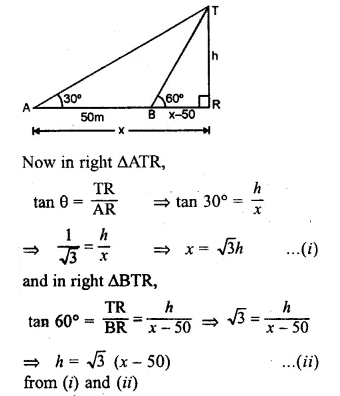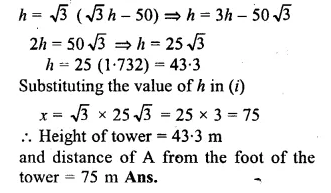Question 2.
An aeroplane 3000 m high passes vertically above another aeroplane at an instant when the angles of elevation of the two aeroplanes from the same point on the ground are 60° and 45° respectively. Find the vertical distance between the two planes.
Solution:
Let A and B are two aeroplanes
and P is a point on the ground such that
angles of elevations from A and B are 60° and 45° respectively.
AC = 3000 m
Let AB = x
∴ BC = 3000 – x
Let PC = y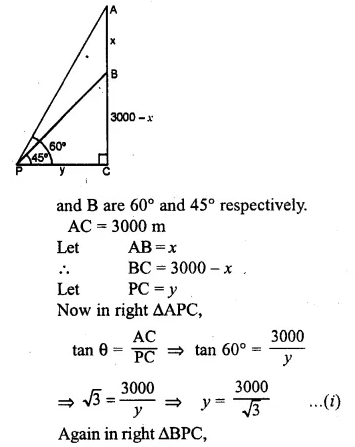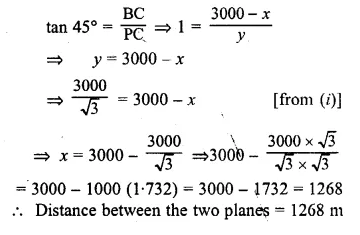Question 3.
A 7m long flagstaff is fixed on the top of a tower. From a point on the ground, the angles of elevation of the top and bottom of the flagstaff are 45° and 36° respectively. Find the height of the tower correct to one place of demical.
Solution:
Let TR be the tower and PT is the flag on it such that PT = 7m
Let TR = h and AR = x
Angles of elevation from P and T are 45° and 36° respectively.
Now in right ∆PAR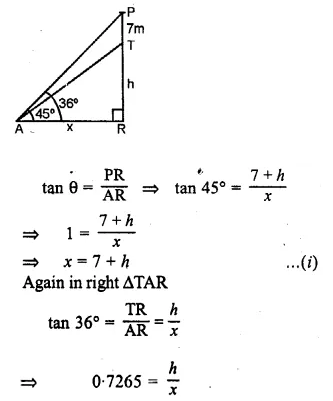Question 4.
A boy 1.6 m tall is 20 m away from a tower and observes that the angle of elevation of the top of the tower is 60°. Find the height of the tower.
Solution:
Let AB be the boy and TR be the tower
∴ AB = 1.6 m
Let TR = h
from A, show AE || BR
∴ ER = AB = 1.6 m
TE = h – 1.6
AE = BR = 20 m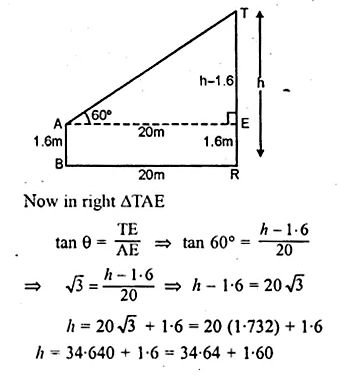h = 36.24
∴ Height of tower = 36.24 m

Question 5.
A boy 1.54 m tall can just see the sun over a wall 3.64 m high which is 2.1 m away from him. Find the angle of elevation of the sun.
Solution:
Let AB be the boy and CD be the wall which is at a distance of 2.1 m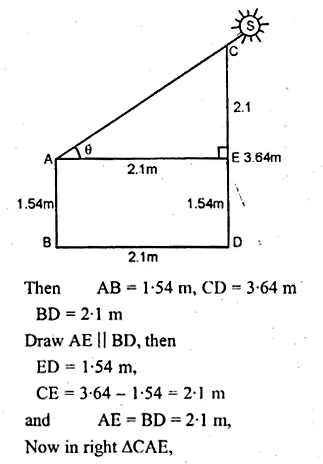Question 6.
In the adjoining figure, the angle of elevation of the top P of a vertical tower from a point X is 60° ; at a point Y, 40 m vertically above X, the angle of elevation is 45°. Find
(i) the height of the tower PQ
(ii) the distance XQ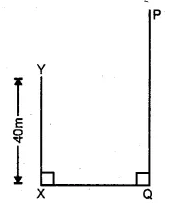Solution:
Let PQ be the tower and let PQ = h
and XQ = YR = y
XY = 40 m
∴ PR = h – 40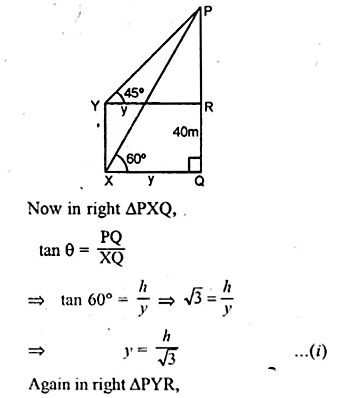Question 7.
An aeroplane is flying horizontally 1 km above the ground is observed at an elevation of 60°. After 10 seconds, its elevation is observed to be 30°. Find the speed of the aeroplane in km/hr.
Solution:
A and D are the two positions of the aeroplane ;
AB is the height and P is the point
∴ AB = 1 km,
Let AD = x and PB = y
and angles of elevation from A and D at point P are 60° and 30° respectively.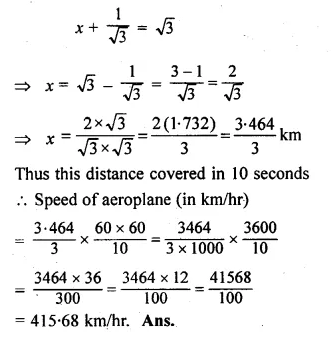Question 8.
A man on the deck of a ship is 16 m above the water level. He observes that the angle of elevation of the top of a cliff is 45° and the angle of depression of the base is 30°. Calculate the distance of the cliff from the ship and the height of the cliff.
Solution:
Let A is the man on the deck of a ship B and CE is the cliff.
AB = 16 m and angle of elevation from the top of the cliff in 45°
and the angle of depression at the base of the cliff is 30°.
Let CE = h, AD = x, then
CD = h – 16, AD = BE = x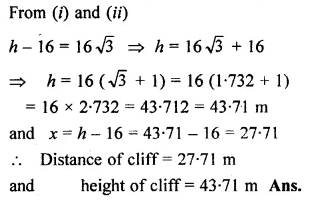Question 9.
There is a small island in between a river 100 metres wide. A tall tree stands on the island. P and Q are points directly opposite to each other on the two banks and in the line with the tree. If the angles of elevation of the top of the tree from P and Q are 30° and 45° respectively, find the height of the tree.
Solution:
The width of the river (PQ) = 100 m.
B is the island and AB is the tree on it.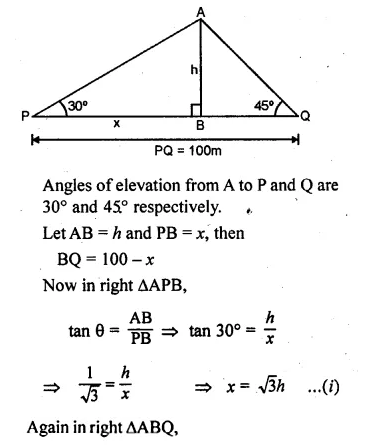Question 10.
A man standing on the deck of the ship which is 20 m above the sea-level, observes the angle of elevation of a bird as 30° and the angle of depression of its reflection in the sea as 60°. Find the height of the bird
Solution:
Let P is the man standing on the deck of a ship
which is 20 m above the sea level and B is the bird.
Now angle of elevation of the bird from P = 30°
and angle of depression from P to the shadow of the bird in the sea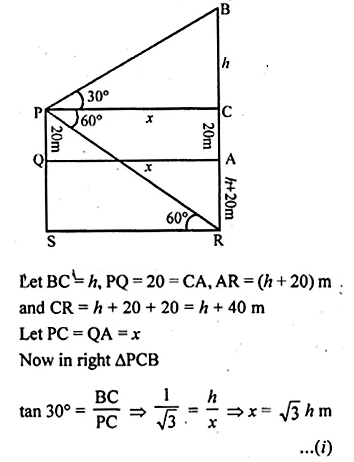We hope the ML Aggarwal Class 10 Solutions for ICSE Maths Chapter 20 Heights and Distances Chapter Test help you. If you have any query regarding ML Aggarwal Class 10 Solutions for ICSE Maths Chapter 20 Heights and Distances Chapter Test, drop a comment below and we will get back to you at the earliest.